Gravity Anomaly Data Reconstruction Based on Improved Interior Point Method in Airborne Gravimetry | OMICS International
Journal of Civil & Environmental Engineering

# Gravity Anomaly Data Reconstruction Based on Improved Interior Point Method in Airborne Gravimetry

 Yapeng Yang* and Meiping Wu Department of Automatic Control, College of Mechatronics and Automation, National University of Defense Technology, Deya Street 109, Changsha 410073, China Corresponding Author : Yapeng Yang Department of Automatic Control College of Mechatronics and Automation National University of Defense Technology Deya Street 109, Changsha 410073, China Tel: +86-137-8618-8163 Fax: +86-731-8457-6305 (ext. 8212) E-mail: [email protected] Received February 10, 2015; Accepted March 24, 2015; Published April 04, 2015 Citation: Yang Y, Wu M (2015) Gravity Anomaly Data Reconstruction Based on Improved Interior Point Method in Airborne Gravimetry. J Civil Environ Eng 5:170. doi:10.4172/2165-784X.1000170 Copyright: © 2015 Yang Y, et al. This is an open-access article distributed under the terms of the Creative Commons Attribution License, which permits unrestricted use, distribution, and reproduction in any medium, provided the original author and source are credited.

Visit for more related articles at Journal of Civil & Environmental Engineering

#### Abstract

Strap-down air borne gravimeter is the core sensor of strap-down gravimetry system. In this paper, A new strap-down airborne gravimeter called SGA-WZ has been developed by the Laboratory of Inertial Technology of the National University of Defense Technology. Precise gravity anomaly data reconstruction is the key technology in airborne gravimetry based on the SGA-WZ. This paper explores a method for large scale precise gravity anomaly data reconstruction using the theory of Compressed Sensing (CS). Based on the CS theory, the gravity anomaly data reconstruction can be transformed into the one-norm convex quadratic program to be solved by several methods such as interior point methods (IPM). This paper presents an improved IPM for performing the large scale gravity anomaly data reconstruction precisely that uses the Preconditioned Conjugate Gradients Algorithm (PCG) to compute the search direction. And then, a flight test has been carried out in China with SGA-WZ. The test results have shown that the PCG-IPM algorithm can reconstruct the large scale gravity anomaly data with higher accuracy than the existing nearest interpolation method in airborne gravimetry.

 Keywords Airborne gravimetry; Gravity anomaly data reconstruction; Compressed sensing; Improved interior point method Introduction The gravity is one of the primary forces on the earth, which affects all the physical events of the earth and it’s near space. Gravity survey is significant in many fields, such as geology, seismology, geophysics, navigation technology, military science, and resource investigation, etc. Airborne gravimetry is an important measuring technology of acquiring the earth gravity. Compared to traditional terrestrial gravimetry and shipborne gravimetry, airborne gravimetry uses the measuring instruments taken on an aircraft, so which can get extensively and precisely well-distributed information of the earth gravity field economically and rapidly . Meanwhile, some special terrains are difficult to access by terrestrial gravimetry and shipborne gravimetry, such as desert, marsh, glacier, mountain and forest, but airborne gravimetry can measure gravity in these areas effectively . Just because of this, airborne gravimetry has become one of the hot research fields in geophysics. Gravity anomaly data reconstruction is an important research topic in airborne gravimetry. Nyquist and Shannon showed that signals can be exactly recovered from a set of uniformly spaced samples taken at the Nyquist rate of twice the highest frequency present in the signal of interest [3,4]. Essentially, airborne gravimetry is a sub-Nyquist sampling method, and then, the precise gravity anomaly data reconstruction becomes a difficult work. In 2006, Candès, Romberg, Tao and Donoho presented a new theory named as CS, which offers the opportunity to perform the gravity anomaly data reconstruction precisely. The theory showed that a signal having a sparse representation can be reconstructed exactly from a small set of linear measurements [5-8]. Taking into account the sparsity of airborne gravimetry by the Discrete Fourier Transform (DFT), the gravity anomaly data reconstruction can be transformed into the one-norm convex quadratic program, which can be solved by standard convex optimization methods such as IPM algorithms. The recently developed computational methods for one-norm convex quadratic program include path-following methods [9,10], bound optimization methods, iterated shrinkage methods , and gradient projection algorithms. This paper mainly describes the PCG-IPM algorithm for performing the large scale gravity anomaly data reconstruction precisely. The method uses the PCG algorithm to compute the search direction . Materials and Methods Compressed sensing is a rapidly growing field that has attracted considerable attention in geophysics, seismology, applied mathematics, and computer science. Especially, reducing the sampling rate and increasing resolution in airborne gravimetry can reduce economic cost, improve survey efficiency, and improve data quality. Let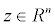be an unknown original gravity anomaly,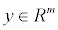is the sampling gravity anomaly, and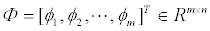is the compressed sensing matrix. If z is sparse in a transform matrix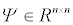and the vectors ɸi are well chosen, the original gravity anomaly can be recovered from a amazingly small number of linear measurements m. And then, based on the theory of CS, the gravity anomaly data reconstruction can be studied by solving a problem of the form: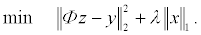(1) Where λ > 0 is the regularization parameter, x is the sparse coefficient matrix, and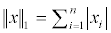is the one-norm of x. When Ψ is invertible, and let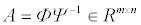, , Equation (1) can be rewritten as: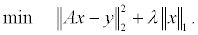(2) This is the one-norm regularized least squares problem. By associating the linear inequality constraints, Equation (2) can be transformed to the convex quadratic program: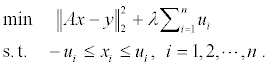(3) The convex quadratic program can be solved by the PCG-IPM algorithm. This paper mainly studies the application of PCG-IPM algorithm in precise gravity anomaly data reconstruction. The method uses the PCG method to compute the search step, therefore, which can reduce the computation time for large scale problems with modest accuracy. Compared with the IPM that uses conjugate gradient methods to compute the search step, the PCG-IPM algorithm is able to solve the gravity anomaly data reconstruction with higher accuracy and smaller computational cost. By associating the dual variable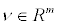, the Lagrange dual of Equation (2) can be formulated as: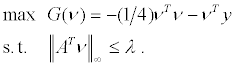(4) This dual problem is a convex optimization problem. Let v* is the solution of Equation (4), then we can say that v* is dual feasible . Meanwhile, if P* is the optimal value of Equation (2), the dual feasible point v* supplies a lower bound of P*, i.e.,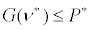. For an arbitrary x of the one-norm regularized least squares problem, an simply computed bound of the sub-optimality x* can be obtained by constructing a dual feasible point: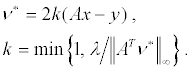(5) Because the point v* is dual feasible, G(ν*) is a lower bound of P*. The difference between G(ν*) and P* is named the duality gap denoted with η :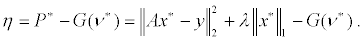(6) where η ≥ 0 . When x* is the optimal solution of Equation (2), the duality gapη = 0 . For the bound constraints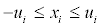in Equation (3), we can define the logarithmic barrier: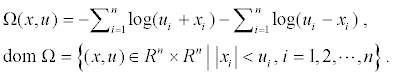(7) And then, Equation (3) can be reformulated to the convex function as: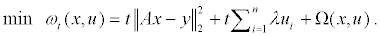(8) As the parameter t varies from 0 to ∞, we can obtain a series of the unique solution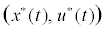of Equation (8). With each point, we can construct the dual feasible variable v* shown in Equation (5). And then, the series of the pointleads to an optimal solution of Equation (2). Generally, the solution of Equation (8) can be obtained with the Newton’s method: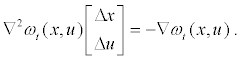(9) The solution of Equation (9) exactly is the search direction (Δx, Δu) . For the gravity anomaly data reconstruction, the PCG algorithm computes the search direction as an approximate solution of Equation (9) [14-16], so it gives a good tradeoff of computational complexity versus the data recovery precision. In Equation (9),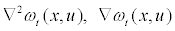can be formulated as: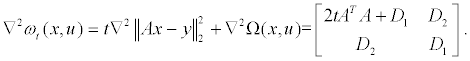(10)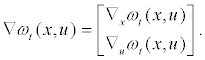(11) With the PCG algorithm, the search direction (Δx, Δu) can be computed approximately [17-19], with a preconditioner: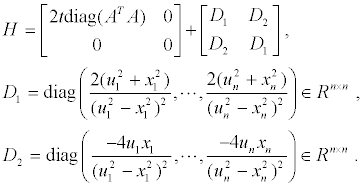(12) where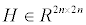is symmetric and positive definite. We can see that Equation (12) is the approximation of Equation (10), so the cost of computing the search direction can be reduced. After obtaining the search direction (Δx, Δu) by using the PCG algorithm, the new point is (x,u) + s(Δx,Δu) , where s>0 is the step size, which can be taken with the backtracking line search method as: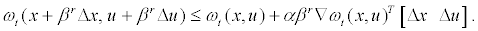(13) If r ≥ 0 is the smallest integer that satisfies Equation (13), the step size is s=βr. Meanwhile, the stopping criterion of the PCG-IPM algorithm can be expressed as: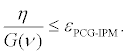(14) Where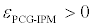is the relative tolerance parameter for the PCG-IPM algorithm. In conclusion, the procedure of PCG-IMP can be carried out by means of the basic steps as follows: Step 1: Initialize the algorithm parameters: t : =1 λ , x : = 0 ,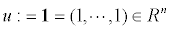Step 2: Compute the approximate solution of Equation (9) to obtain the search direction (Δx, Δu) , by using the PCG algorithm. Step 3: Evaluate the step size s with the backtracking line search method, and update the new point (x,u) + s(Δx,Δu) . Step 4: Construct the dual feasible variable v* shown in Equation (5), and compute the dual gap η shown in Equation (6). Step 5: Update the parameter t. Step 6: If the stopping criterion shown in Equation (14) is met, the PCG-IPM algorithm will come to the end. Else, return to Step 2. Step 7: Obtain the estimate for the unknown gravity anomaly: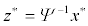Test Results and Discussions From April 2010 to May 2010, the flight test was carried out in China. Among the eight flights in the whole flight test, the first and second flights were repeated lines. The other six flights were grid flights consisting of three flights of survey lines and three flights of control lines. In the test, we used the strap-down airborne gravimetry system, the core of which is strap-down airborne gravimeter. Because the strapdown airborne gravimeter has so many advantages of high reliability, low cost, unattended operation, and so on, it attracts broad attention of the researchers and develops rapidly . In this paper, the Laboratory of Inertial Technology of the National University of Defense Technology has developed a strap-down airborne scalar gravimeter independently, as Figure 1 shows, which is the first home-grown system in China called SGA-WZ. In this paper, the SGA-WZ can be divided into two main components: the strap-down inertial navigation system [21,22] and the triad of accelerometers (Figure 2). The strap-down inertial navigation system is used mainly for measuring the specific force and the attiude of the aircraft. The accelerometers have a stability of ± 0.2 mGal, scale factor uncertainty of ± 3 ppm and random noise of ± 5 mGal. The gyroscopes have a stability of ± 0.004°/h and random noise of ± 0.002°/√h .To evaluate the PCG-IPM algorithm performance and the gravity anomaly data reconstruction precision, this study defines the performance indices with the standard deviation and SNR. SNR can be calculated by: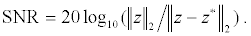(15) Due to space limitations, this paper mainly analyzes the first flight denoted by F401, which consists of 6 lines. In this study, the algorithm parameters of PCG-IPM were set as: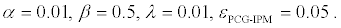(16) And the compressed measurement number was set as m=1618. Figure 3 shows the reconstruction results of line 1 in F401 by the PCG-IPM algorithm. The comparisons between the original gravity anomaly and reconstruction gravity anomaly of line 1 in F401 are shown in Figure 3a. In this subgraph, the red dashed denotes the reconstruction gravity anomaly, the blue solid denotes the original gravity anomaly, and the two curves almost overlap each other if it doesn’t take the boundary effect into account. The reconstruction error of line 1 in F401 is shown in Figure 3b. Without regard to the boundary effect, the maximum reconstruction error of line 1 in F401 is 0.108 mGal, the minimum reconstruction error of line 1 in F401 is -0.132 mGal, and the standard deviation of reconstruction error of line 1 in F401 is 0.023 mGal. In practice, if the boundary data can be abandoned in the post-mission analysis, the boundary effect can also be ignored.As a consequence, Figure 3 demonstrates that the reconstruction results from the PCG-IPM algorithm approximate the original gravity anomaly very well and the reconstruction error is also very small. As mentioned above, the compressed measurement number was set as m =1618 , i.e., the compressed sampling rate was approximately 52%. These results convincingly show that the PCGIPM algorithm can reconstruct the gravity anomaly data with higher accuracy and fewer measurements. To contrast the gravity anomaly data reconstruction precision of the PCG-IPM algorithm, the paper also made the gravity anomaly data reconstruction with the existing Nearest Interpolation Method (NIP), which is one of the commonly used methodologies for data reconstruction in airborne gravimetry. Figure 4 shows the reconstruction results of line 1 in F401 by the NIP algorithm. The comparisons between the original gravity anomaly and reconstruction gravity anomaly of line 1 in F401 are shown in Figure 4a. Be similar to Figure 3a, the red dashed denotes the reconstruction gravity anomaly, and the blue solid denotes the original gravity anomaly. The reconstruction error of line 1 in F401 is shown in Figure 4b. The maximum reconstruction error of line 1 in F401 is 0.289 mGal, the minimum reconstruction error of line 1 in F401 is -0.293 mGal, and the standard deviation of reconstruction error of line 1 in F401 is 0.11 mGal. As a consequence, Figures 4 demonstrates that there is not the boundary effect existing in the reconstruction results of the NIP algorithm, but the gravity anomaly data reconstruction precision of the NIP algorithm is inferior to the corresponding one of the PCG-IPM algorithm. The comparisons of the algorithm performance between the PCG-IPM and NIP algorithm for F401 are shown in Table 1. In Table 1, taking an average, the standard deviation of reconstruction error and SNR of the PCG-IPM algorithm in F401 are 0.024 mGal and 58.277 dB, respectively. Correspondingly, the standard deviation of reconstruction error and SNR of the NIP algorithm are 0.109 mGal and 45.283 dB, respectively. Conclusion Strap-down airborne gravimeter is the core sensor of strap-down gravimetry system. This paper explores a method for large scale precise gravity anomaly data reconstruction based on the strap-down airborne gravimeter. Precise gravity anomaly data reconstruction is the key technology in airborne gravimetry. In this paper, our work can be summarized as follows: (1) The strap-down airborne gravimeter (SGA-WZ) mentioned in this paper is the first system with this type in China. The system is an inexpensive, robust, flexible tool to carry out airborne gravimetry. (2) Based on the theory of CS, the paper presents the PCG-IPM algorithm applied to perform the large scale gravity anomaly data reconstruction precisely. (3) A flight test was carried out in China. By the comparison between the PCG-IPM algorithm and the NIP algorithm, the each performance index from the PCG-IPM algorithm is superior to the corresponding one from the NIP algorithm. These results convincingly show that the PCG-IPM algorithm can reconstruct the gravity anomaly data with higher accuracy and fewer measurements. However, the boundary effect exists in the result of gravity anomaly data reconstruction with the PCG-IPM algorithm. Although we can abandon the boundary data, that will result in the waste of data and increase the survey costs. This problem should be solved in the future researchers. Conflict of Interests The authors declare that there is no conflict of interests regarding the publication of this paper. Acknowledgment This work was supported by the National High Technology Research and Development Program of China (SS2013AA060402). The authors would like to thank the anonymous reviewers for their helpful comments which have improved the quality of the paper. References Nettleton LL, LaCoste L, Harrison JC (1960)“Test of an airborne gravity meter”.Geophys 25: 181-202. Wang LH, Zhang CD, Wang JQ(2008) “Mathematical models and accuracy evaluation for the airborne gravimetry”.J Geom Sci Technol 25: 68-71. Shannon C (1949) “Communication in the presence of noise.” Proc Inst Radio Engineers 37: 10-21. Nyquist H(1928) “Certain topics in telegraph transmission theory.” Trans AIEE47: 617-644. Candès E, Romberg J (2006) “Quantitative robust uncertainty principles and optimally sparse decompositions.” Found Comput Math 6: 227-254. Donoho DL (2006) “Compressed sensing.” IEEE Trans Inform Theor 52: 1289-1306. Candès E, Romberg J, Tao T (2006) “Robust uncertainty principles: Exact signal reconstruction from highly incomplete frequency information.” IEEE Trans Inform Theor52: 489-509. Candès E, Tao T (2006) “Near optimal signal recovery from random projections:Universal encoding strategies.” IEEE Trans Inform Theor52: 5406-5425. Efron B, Hastie T, Johnstone I, Tibshirani R (2004) “Least angle regression.” Ann Stat 32: 407-499. Osborne M, Presnell B, Turlach B (2000) “A new approach to variable selection in least squares problems.” IMA J Numer Anal20: 389-404 Daubechies I, Defrise M, Demol C (2004) “An iterative thresholding algorithm for linear inverse problems with a sparsity constraint.” Commun PurAppl Math57: 1413-1541. Kim SJ, Koh K, Lustig M, Boyd S, Gorinevsky D, et al. (2007) “An Interior-Point Method for Large-Scale L1-Regularized Least Squares.” IEEE J Selected Top Signal Process1: 606-617. Boyd S, Vandenberghe L(2004) Convex Optimization. Cambridge University Press, New York, USA Johnson C, Seidel J, Sofer A (2000) “Interior point methodology for 3-D PET reconstruction.” IEEE Trans Med Imag 19: 271-285. Koh K, Kim SJ, Boyd S (2007) “An interior-point method for L1-regularized logistic regression.” J Mach Learning Res8:1519-1555. Vandenberghe L, Boyd S (1995) “A primal-dual potential reduction method for problems involving matrix inequalities.” Math Program 69: 205-236. Demmel J (1997)Applied Numerical Linear Algebra. Cambridge University Press, New York, USA. Nocedal J, Wright S(1999) Numerical Optimization.Springer Series in Operations Research, New York, USA Eldar YC, Kutyniok G (2012) Compressed Sensing: Theory and Applications. Cambridge University Press, New York, USA. Xiong SQ, Zhou XH, Guo ZH, Zhou JX (2010) Theory, Method and Application of the Airborne Gravity Prospecting. Geology Press, Beijing, China. Huang YM, Olesen AV, Wu MP, Zhang KD (2012) “SGA-WZ: A new strapdown airborne gravimeter.” Sensors 12: 9336-9348. Cai SK, Wu MP, Zhang KD, Cao JL, TuoJH, et al. (2013) “The first airborne scalar gravimetry system based on SINS/DGPS in China.” Sci China Earth Sci56: 2198-2208.

#### Tables and Figures at a glanceTable 1

#### Figures at a glance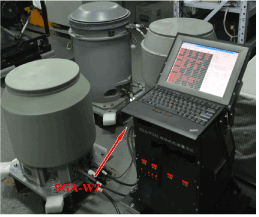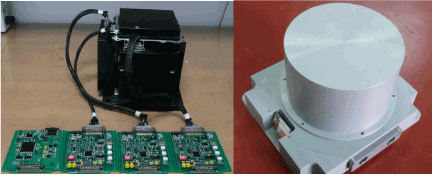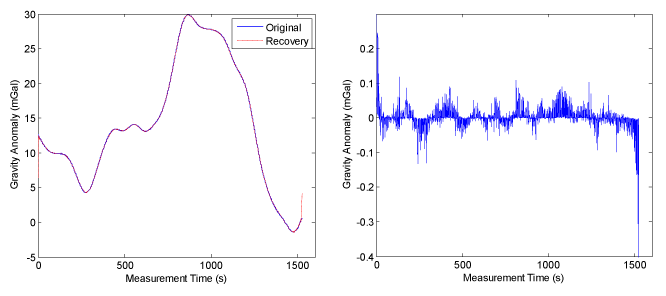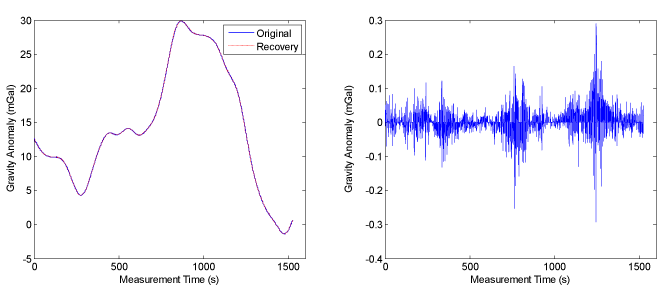Figure 1 Figure 2 Figure 3 Figure 4
Select your language of interest to view the total content in your interested language

### Article Usage

• Total views: 12251
• [From(publication date):
April-2015 - Feb 21, 2020]
• Breakdown by view type
• HTML page views : 8426Can't read the image? click here to refresh